Activate JavaScript to enable computations!

# Pulsed Power Toolbox

This is the start of a collection of Pulsed Power related formulas and calculators that I found usefull. However, whenever I could find resources on the internet already, I tried to refer to them rather than to re-invent the wheel. Consequently you might also find some of the links provided helpful.

## Calculation of Rise Times and Peak Currents for RCL-Circuits*)

(unless otherwise noted use SI-units in all presented formulas)

*) Of course no guarantee is given for the accuracy of the listed formulaes or the calculators although I tried my best to be careful. Please let me know when you find any mistakes.

RCL-Circuit

RCL-circuits (also often called RLC-circuits) are often encounterd in the description of the discharge characteristic of a pulse generator, especially for capacitors and including Marx-bank generators. The challenge is to estimate peak current (and voltage) and how fast they could be achieved. Conversely, a comparison with measured outputs allows in particular estimations on system-inductances. The tool will help to estimate current peaks and associated rise times. Voltage peaks can then be derived from the values of respective circuit elements R and L.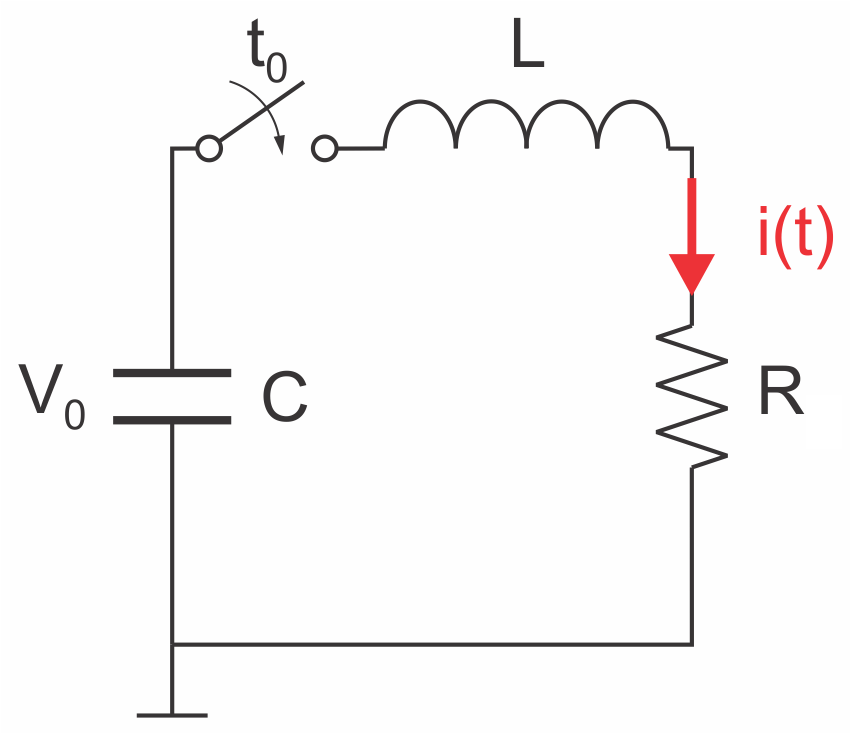Current waveforms for underdamped, critically damped and overdamped parameters: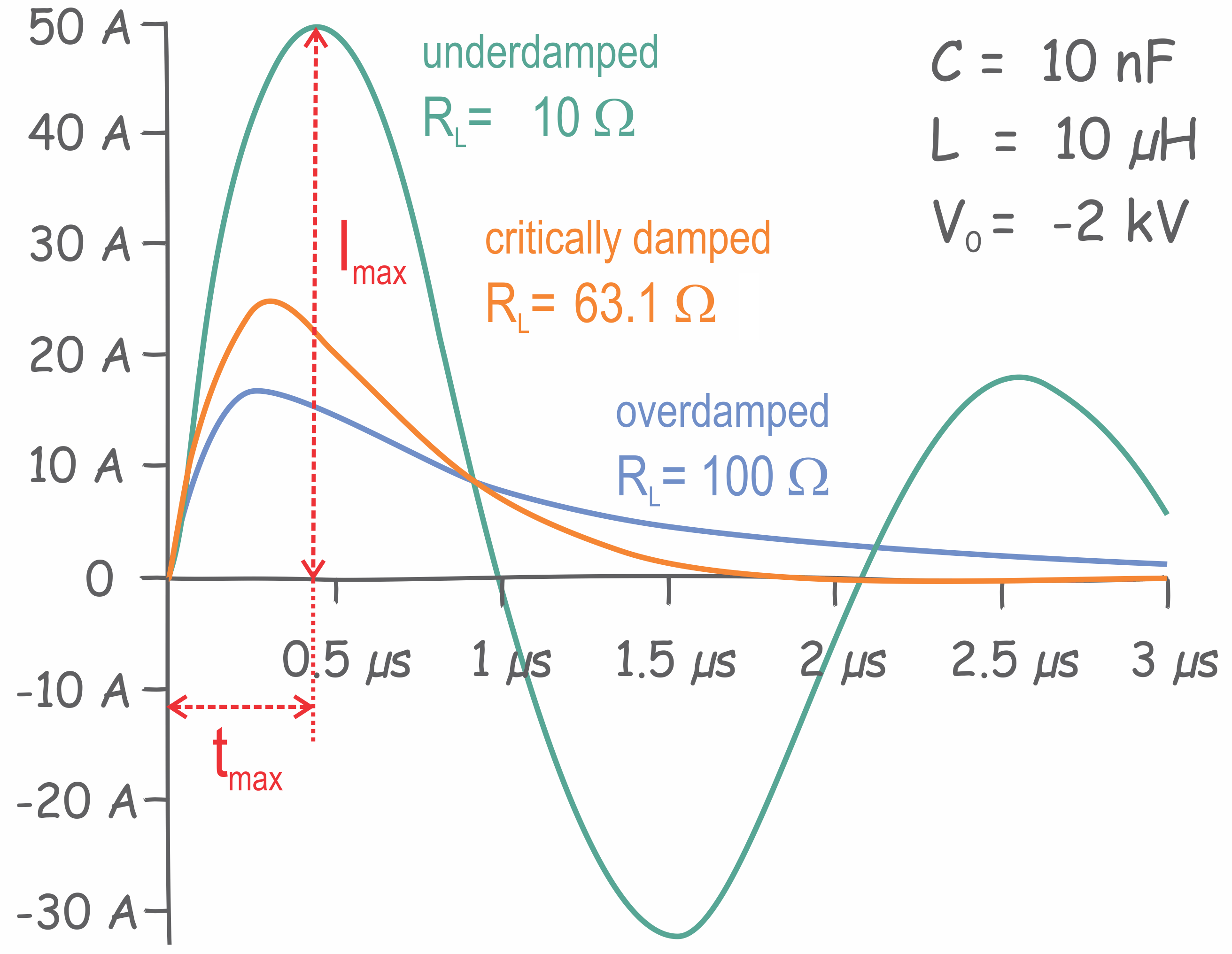Underdamped solution (δ < ω0):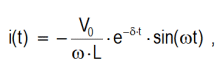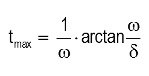Critically damped solution (δ = ω0):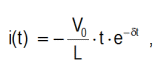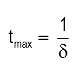Overdamped solution (δ > ω0):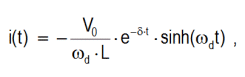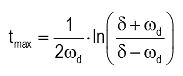Solutions for the transient response when discharging a capacitance, C, through an inductance, L, and load resistance, R, can be found in respective textbooks. In short: from Kirchof's law for the voltage along a closed loop, a second order differental equation can be derived. Depending on the solution of the characteristic equation, the response can be described by either an underdamped, a critically damped or an overdamped solution.

Kirchhof's law for voltage loops: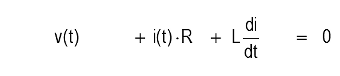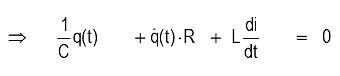Characteristic equation: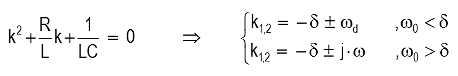with resonance frequency (undamped natural frequency):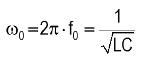and damping attenuation: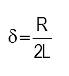and damped natural frequency: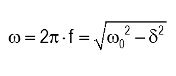or the "frequency" for the overdamped response: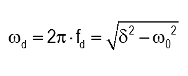Approach to Derive Solutions

Ansatz: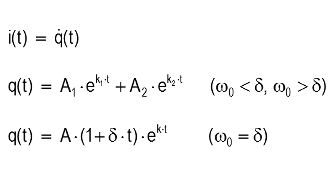Boundary conditions: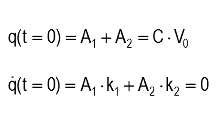R = Ω C = μF L = μH V0 = kV